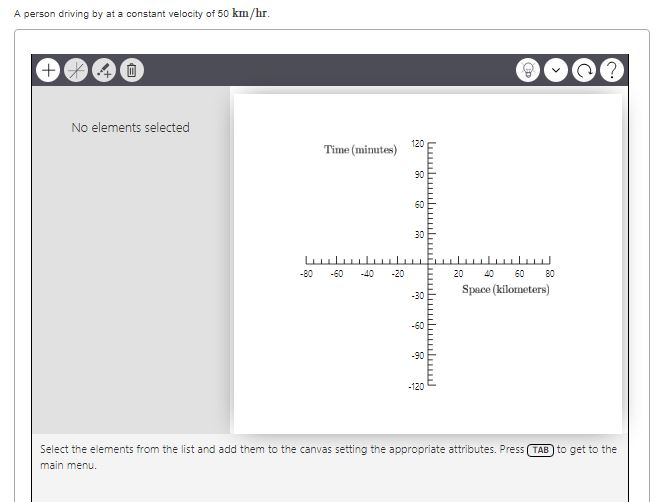# Problem: Make a spacetime diagram and draw worldlines for each of the following situationsA person driving by at a constant velocity of 50 km/hr.

###### FREE Expert Solution

In this problem, we are required to apply the relationship between distance, speed and time.

$\overline{){\mathbf{d}}{\mathbf{=}}{\mathbf{v}}{\mathbf{·}}{\mathbf{t}}}$

100% (115 ratings)###### Problem Details

Make a spacetime diagram and draw worldlines for each of the following situations

A person driving by at a constant velocity of 50 km/hr.